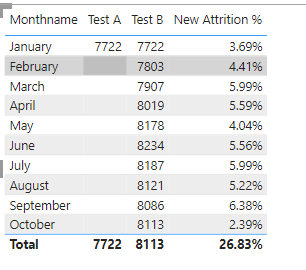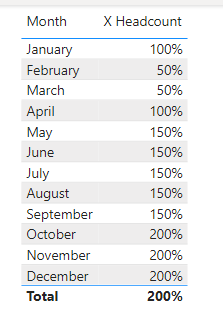cancel
Showing results for
Did you mean:Helper I

Hi Team,

I'm unable to get the headcount for the required condition.

1. Since we do not have data for 12 months yet, then we have to calculate average headcount to be = (Headcount of Jan +Headcount of current month)/2This is my formula

var x = CALCULATE(DISTINCTCOUNT(EIR[Employee ID]),ALL(EIR[Date Relation]),EIR[Date Relation] = DATE(2022,01,27))

var y = CALCULATE(DISTINCTCOUNT(EIR[Employee ID]),EIR[Date Relation] = MAX(EIR[Date Relation]))

var Result = (x + y)/2
return
Result

3 REPLIES 3Community Support

Hi @Rajkumar07 ,

Please new a calendar table first, like:

``````Calendar =
CALENDAR(DATE(2022,1,1),DATE(2022,12,31)),
"Month",FORMAT([Date],"mmmm"),
"Month Number",MONTH([Date])
)``````

Relationship:then new a measure:

``````X Headcount =
VAR __MONTH =
MAX ( 'Calendar'[Month Number] )
VAR __X =
CALCULATE (
COUNTROWS ( 'StarterTable' ),
MONTH ( 'StarterTable'[Date Relation] ) <= 1
)
VAR __Y =
CALCULATE (
COUNTROWS ( 'StarterTable' ),
MONTH ( 'StarterTable'[Date Relation] ) <= __MONTH
)
- CALCULATE (
COUNTROWS ( 'LeaverTable' ),
MONTH ( 'LeaverTable'[Date Relation] ) <= __MONTH
)
VAR __RESULT =
DIVIDE ( __X + __Y, 2 )
RETURN
__RESULT``````

apply the measure with 'calendar'[month] to the table visual, resilt:The PBIX file is attached for reference.

Best Regards,
Gao

Community Support Team

If there is any post helps, then please consider Accept it as the solution  to help the other members find it more quickly. If I misunderstand your needs or you still have problems on it, please feel free to let us know. Thanks a lot!

How to get your questions answered quickly -- How to provide sample dataSolution Sage

Hi, can you please past some sample data of your table EIR?Helper I

Hi,

This is how look my sample dataI have a sperate Starter and Leaver Table this also looking like same.

Here is my requirement have to find the Attrition %
I'm already having the Leavers count which numerator value. I need to find the Denominator.
Like my first request.Announcements#### Exclusive opportunity for Women!

Join us for a free, hands-on Microsoft workshop led by women trainers for women where you will learn how to build a Dashboard in a Day!#### Power Platform Conference-Power BI and Fabric Sessions

Join us Oct 1 - 6 in Las Vegas for the Microsoft Power Platform Conference.Top Solution Authors
Top Kudoed Authors
Users online (1,946)